# Area Model - Definition with Examples

The Complete K-5 Math Learning Program Built for Your Child

• 30 Million Kids

Loved by kids and parent worldwide

• 50,000 Schools

Trusted by teachers across schools

• Comprehensive Curriculum

Aligned to Common Core

What is Area Model?

In mathematics, an area model is a rectangular diagram or model used for multiplication and division problems, in which the factors or the quotient and divisor define the length and width of the rectangle.

We can break one large area of the rectangle into several smaller boxes, using number bonds, to make the calculation easier. Then we add to get the area of the whole, which is the product or quotient.

To multiply two 2-digit numbers, using the area model, follow the given steps:

• Write the multiplicands in expanded form as tens and ones.

For example, 27 as 20 and 7, and 35 as 30 and 5.

• Draw a 2 × 2 grid, that is, a box with 2 rows and 2 columns.

• Write the terms of one of the multiplicands on the top of the grid (box). One on the top of each cell.

• On the left of the grid, write the terms of the other multiplicand. One on the side of each cell.

• Write the product of the number on the tens in the first cell. Then write the product of the tens and ones in the second and third cell. Write the product of the ones in the fourth cell.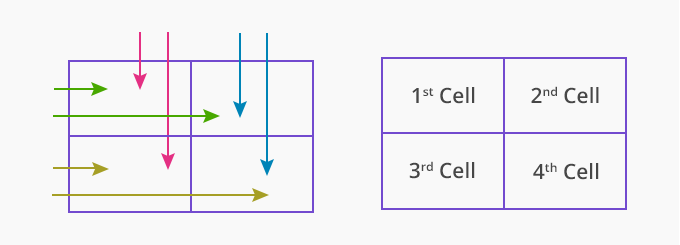• Finally, add all the partial products to get the final product.

Here, for example, the area model has been used to multiply 27 and 35.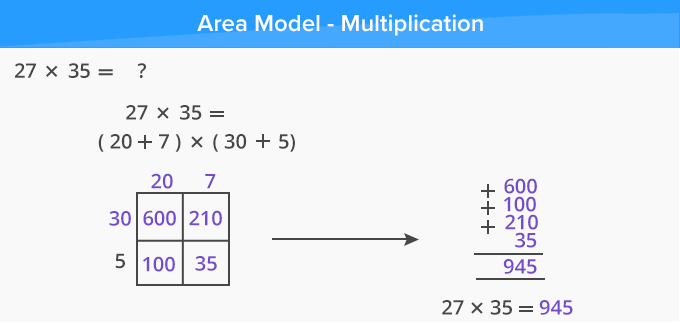Let us see how to find the product of 3-digit number by a 2-digit number using the area model.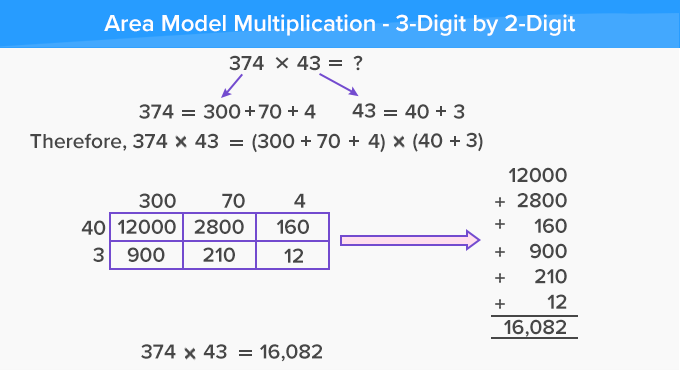Let us now use the area model for the division. Here, we divide 825 by 5.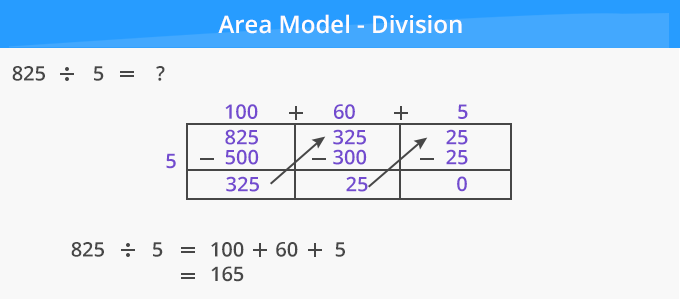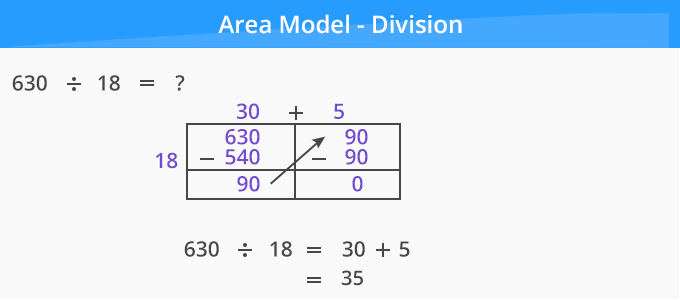Fun Facts The area model is also known as the box model. The area model of solving multiplication and division problems is derived from the concept of finding the area of a rectangle. Area of a rectangle = Length × Width.

Won Numerous Awards & Honors